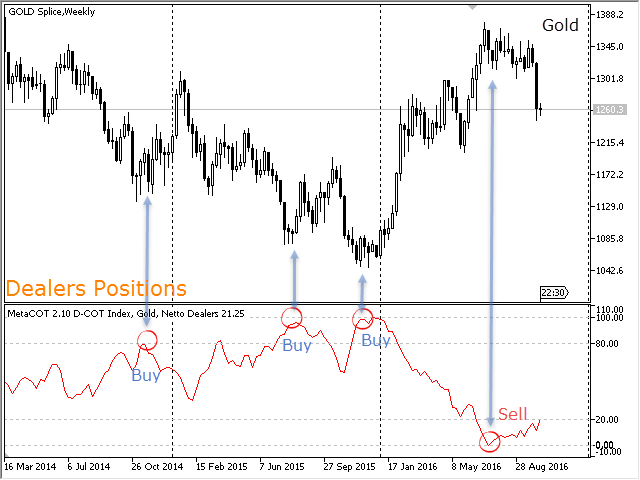# Commitment of Traders (COT) Indicator for ThinkorSwim

#### Jerald643

##### New member
If anyone is familiar with the famous trader Larry Williams you will know he uses the COT (Commitment of Traders) data. It’s free public data. I was wondering if anyone has tried to write a script for that COT indicator?

Does anyone know of COT data is available on Ameritrade's platform? If not, with thinkscript, can I use external data or write a provider/connector for external data?Solution
You can try this for ThinkorSwim:

Code:
``````input n = 38;

def o = open;
def h = high;
def l = low;
def c = close;

# TrueHigh = Highest of High or Close
def TrueHigh = Max(high, close);
# TrueLow = Lowest of Low or Close
def TrueLow = Min(low, close);
def OI = if isNaN(open_interest)
then OI
else open_interest;
def poiv = sum (OI * (close-close / (Truehigh - Truelow)) + onBalanceVolume());
plot values = (lowest(poiv, n)/highest(poiv,n));
# End Code COT proxy``````

Of course, you can access all the COT info you like at Barchart...

#### gapcap1

##### New member
You can try this for ThinkorSwim:

Code:
``````input n = 38;

def o = open;
def h = high;
def l = low;
def c = close;

# TrueHigh = Highest of High or Close
def TrueHigh = Max(high, close);
# TrueLow = Lowest of Low or Close
def TrueLow = Min(low, close);
def OI = if isNaN(open_interest)
then OI
else open_interest;
def poiv = sum (OI * (close-close / (Truehigh - Truelow)) + onBalanceVolume());
plot values = (lowest(poiv, n)/highest(poiv,n));
•